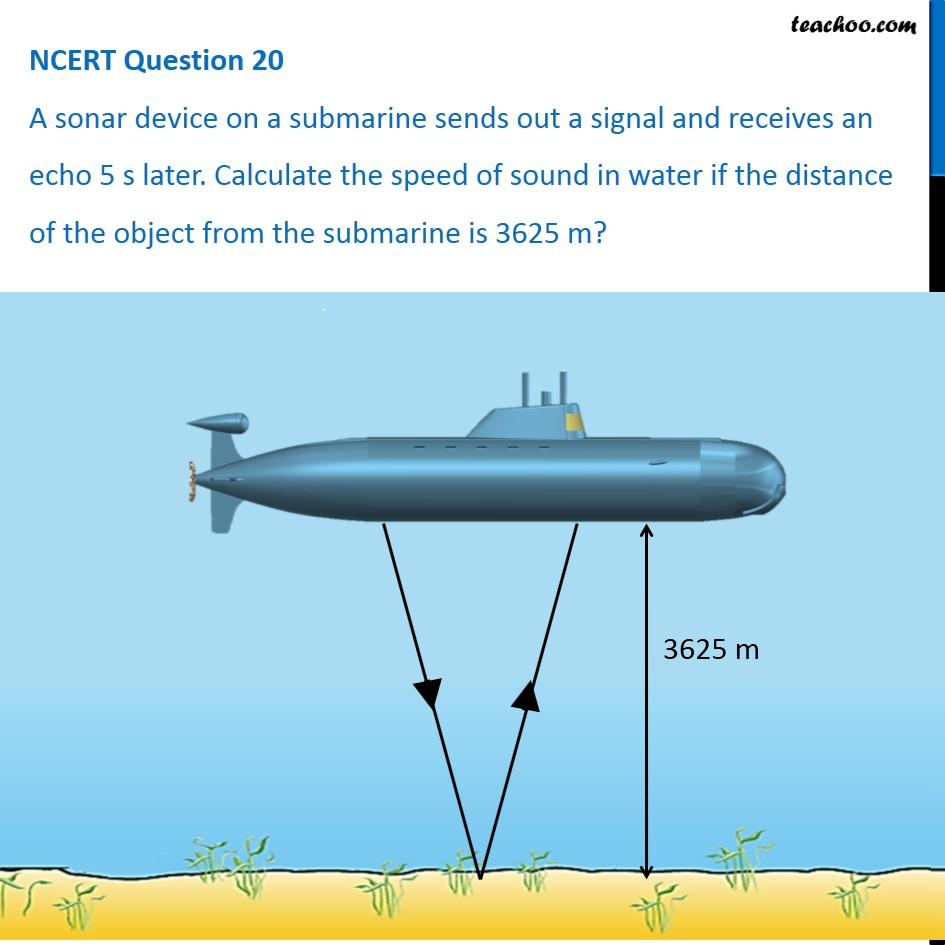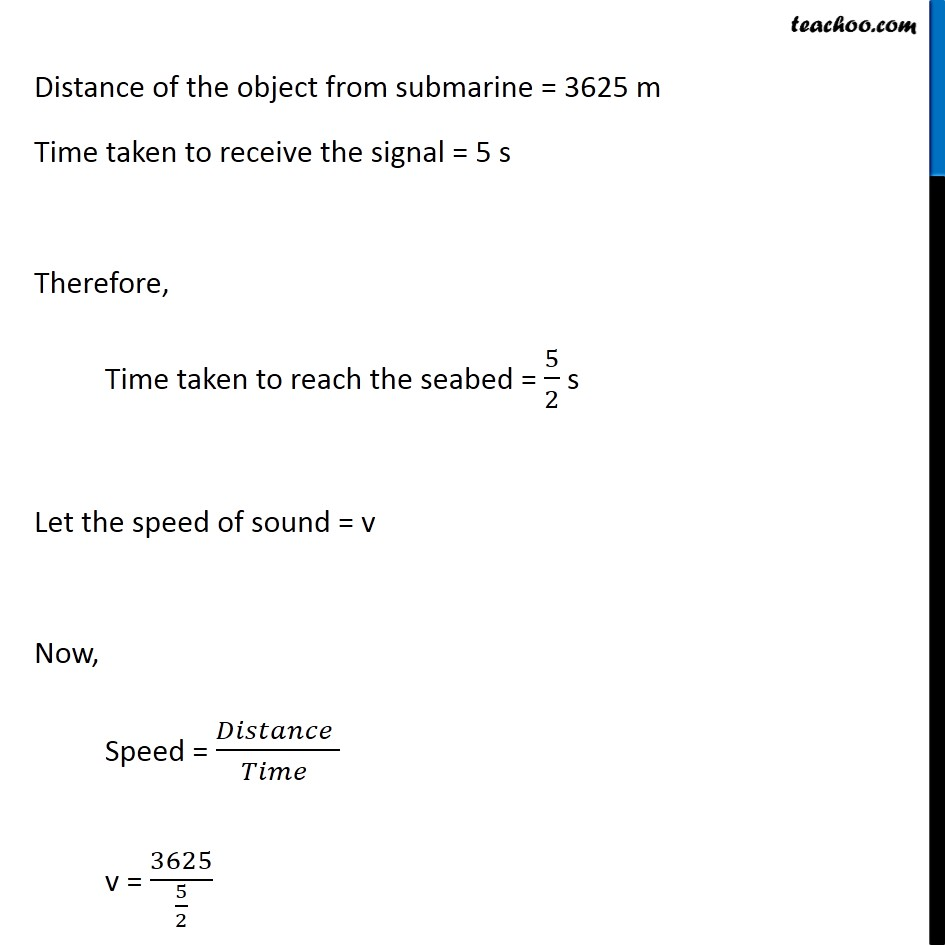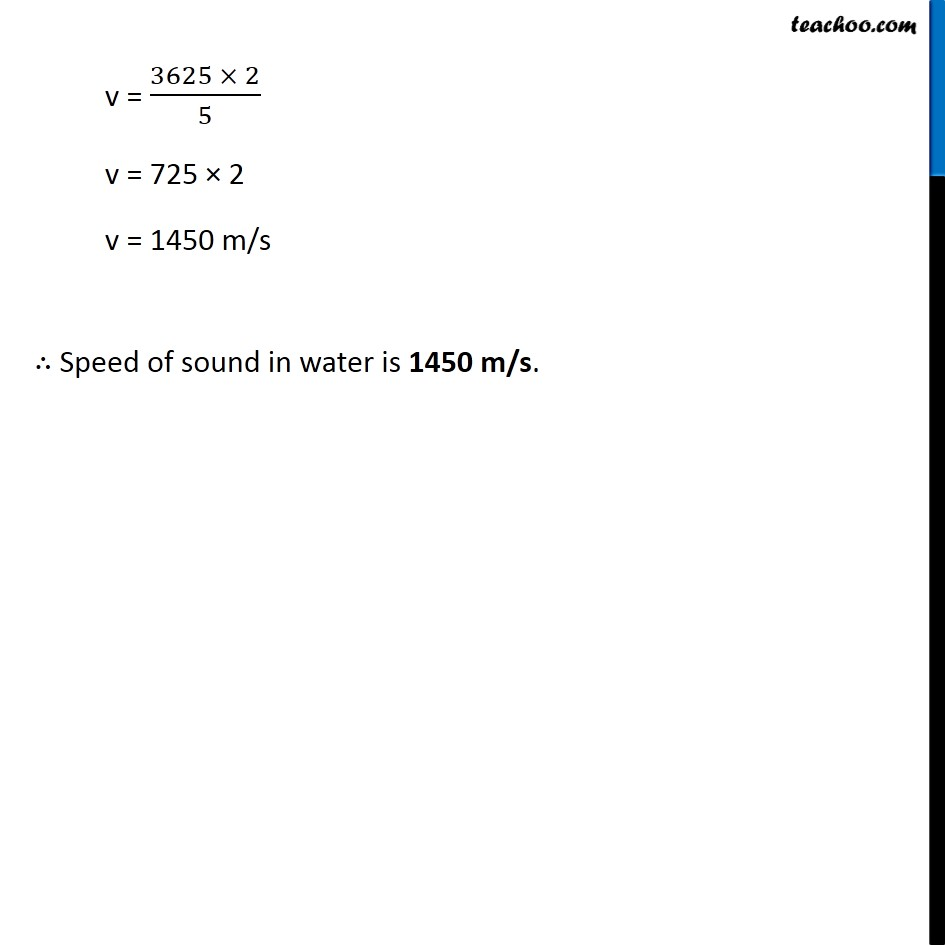NCERT Questions

Class 9
Chapter 12 Class 9 - SoundLearn in your speed, with individual attention - Teachoo Maths 1-on-1 Class

### Transcript

NCERT Question 20 A sonar device on a submarine sends out a signal and receives an echo 5 s later. Calculate the speed of sound in water if the distance of the object from the submarine is 3625 m? Distance of the object from submarine = 3625 m Time taken to receive the signal = 5 s Therefore, Time taken to reach the seabed = 5/2 s Let the speed of sound = v Now, Speed = (𝐷𝑖𝑠𝑡𝑎𝑛𝑐𝑒 )/(𝑇𝑖𝑚𝑒 ) v = 3625/(5/2) v = (3625 × 2)/5 v = 725 × 2 v = 1450 m/s ∴ Speed of sound in water is 1450 m/s.